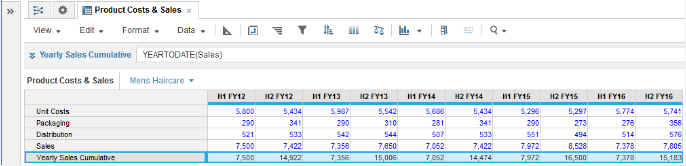Returns cumulative sums across a year time span from a single numeric parameter as source then resets.

## Syntax

`YEARTODATE(x)`

where:

• x: Line item holding the value to cumulate.

## Format

Input Format Output Format

x: Number

Matches the data format used by argument x

## Arguments

The function uses the following arguments:

• x: Number: Numeric line item, property, or expression.

## Constraints

The function has the following constraints:

• Time Scale of source must be Day, Week, Month, Quarter, or Half-Year.
• Cannot be used if model Calendar Type is Weeks: General.

## Excel equivalent

• No Excel equivalent

## Example

YEARTODATE is used to show cumulative sales figures for each year period for FY12 through FY16.

`YEARTODATE(Sales)        `